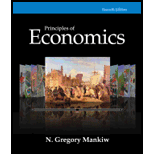# The Federal Reserve expands the money supply by 5 percent. a. Use the theory of liquidity preference to illustrate in a graph the impact of this policy on the interest rate. b. Use the model of aggregate demand and aggregate supply to illustrate the impact of this change in the interest rate on output and the price level in the short run. c. When the economy makes the transition from its short-run equilibrium to its new long-run equilibrium, what will happen to the price level? d. How will this change in the price level affect the demand for money and the equilibrium interest rate? e. Is this analysis consistent with the proposition that money has real effects in the short run but is neutral in the long run?### Principles of Economics, 7th Editi...

7th Edition
N. Gregory Mankiw
Publisher: Cengage Learning
ISBN: 9781285165875

#### Solutions

Chapter
Section### Principles of Economics, 7th Editi...

7th Edition
N. Gregory Mankiw
Publisher: Cengage Learning
ISBN: 9781285165875
Chapter 34, Problem 2PA
Textbook Problem
1 views

## The Federal Reserve expands the money supply by 5 percent.a. Use the theory of liquidity preference to illustrate in a graph the impact of this policy on the interest rate.b. Use the model of aggregate demand and aggregate supply to illustrate the impact of this change in the interest rate on output and the price level in the short run.c. When the economy makes the transition from its short-run equilibrium to its new long-run equilibrium, what will happen to the price level?d. How will this change in the price level affect the demand for money and the equilibrium interest rate?e. Is this analysis consistent with the proposition that money has real effects in the short run but is neutral in the long run?

(a):

To determine

Liquidity preference theory to explain the impact of the policy.

### Explanation of Solution

Figure 1 illustrates the impact of increasing money supply on equilibrium position.

Figure 1 illustrates the impact of the expansionary policy on the interest rate. The horizontal axis in figure 1 represents the quantity of money demanded and supplied, and the vertical axis denotes the interest rate. By the theory of liquidity preference, an increase in the money supply shifts the money–supply curve to the right causing the equilibrium interest rate to decline as shown in Figure 1...

(b):

To determine

Impact of change in interest rate on output and price.

(c):

To determine

Change in price due to change the short run equilibrium to long run equilibrium.

(d):

To determine

Impact of change in the price level on demand for money and interest rate.

(e):

To determine

Real effect of money

### Still sussing out bartleby?

Check out a sample textbook solution.

See a sample solution

#### The Solution to Your Study Problems

Bartleby provides explanations to thousands of textbook problems written by our experts, many with advanced degrees!

Get Started

Find more solutions based on key concepts
What is inflation and what causes it?

Brief Principles of Macroeconomics (MindTap Course List)

Why should policymakers think about incentives?

Principles of Microeconomics (MindTap Course List)

What is accounting entity?

Cornerstones of Financial Accounting

Briefly explain what is meant by the term efficiency continuum.

Fundamentals of Financial Management, Concise Edition (with Thomson ONE - Business School Edition, 1 term (6 months) Printed Access Card) (MindTap Course List)

Who issues a credit memorandum and why?

College Accounting (Book Only): A Career Approach

Could the United States ever experience another Great Depression? Why or why not?

Economics: Private and Public Choice (MindTap Course List)